## DEV Community is a community of 642,334 amazing developers

We're a place where coders share, stay up-to-date and grow their careers.

loading...

# Understanding Neural Networks: What, How and Why?Eugenia
I'm a passionate data scientist who loves new challenges.
Originally published at towardsdatascience.com on ・12 min read#### Unraveling the black box

Neural networks is one of the most powerful and widely used algorithms when it comes to the subfield of machine learning called deep learning. At first look, neural networks may seem a black box; an input layer gets the data into the “hidden layers” and after a magic trick we can see the information provided by the output layer. However, understanding what the hidden layers are doing is the key step to neural network implementation and optimization.

In our path to understand neural networks, we are going to answer three questions: What, How and Why?

#### WHAT is a Neural Network?

The neural networks that we are going to considered are strictly called artificial neural networks, and as the name suggests, are based on what science knows about the human brain’s structure and function.

Briefly, a neural network is defined as a computing system that consist of a number of simple but highly interconnected elements or nodes, called ‘neurons’, which are organized in layers which process information using dynamic state responses to external inputs. This algorithm is extremely useful, as we will explain later, in finding patterns that are too complex for being manually extracted and taught to recognize to the machine. In the context of this structure, patterns are introduced to the neural network by the input layer that has one neuron for each component present in the input data and is communicated to one or more hidden layers present in the network; called ‘hidden’ only due to the fact that they do not constitute the input or output layer. It is in the hidden layers where all the processing actually happens through a system of connections characterized by weights and biases (commonly referred as W and b) : the input is received, the neuron calculate a weighted sum adding also the bias and according to the result and a pre-set activation function (most common one is sigmoid, σ, even though it almost not used anymore and there are better ones like ReLu_), it decides whether it should be ‘fired’ or activated. Afterwards, the neuron transmit the information downstream to other connected neurons in a process called ‘_forward pass’. At the end of this process, the last hidden layer is linked to the output layer which has one neuron for each possible desired output.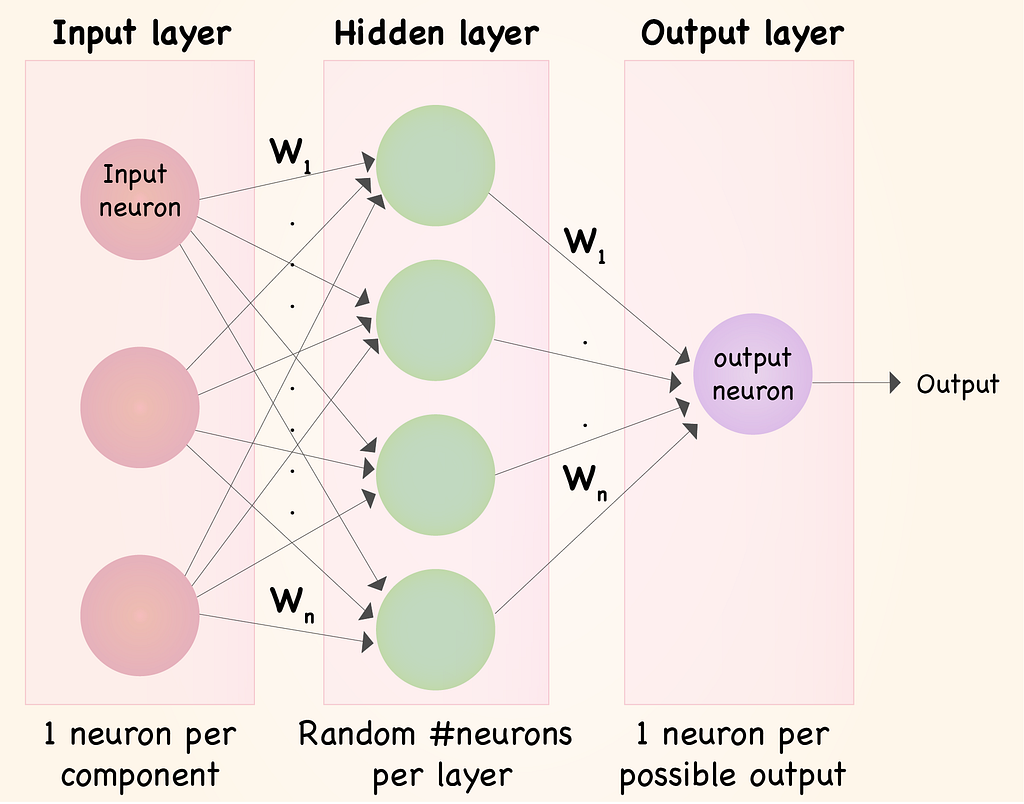#### HOW does a Neural Network work?

Now that we have an idea on how the basic structure of a Neural Network look likes, we will go ahead and explain how it works. In order to do so, we need to explain the different type of neurons that we can include in our network.

The first type of neuron that we are going to explain is Perceptron . Even though its use has decayed today, understanding how they work will give us a good clue about how more modern neurons function.

A perceptron uses a function to learn a binary classifier by mapping a vector of binary variables to a single binary output and it can also be used in supervised learning. In this context, the perceptron follows these steps:

1. Multiply all the inputs by their weights w , real numbers that express how important the corresponding inputs are to the output,
2. Add them together referred as weighted sum: ∑ wj xj ,
3. Apply the activation function , in other words, determine whether the weighted sum is greater than a threshold value, where -threshold is equivalent to bias, and assign 1 or less and assign 0 as an output_._

We can also write the perceptron function in the following terms: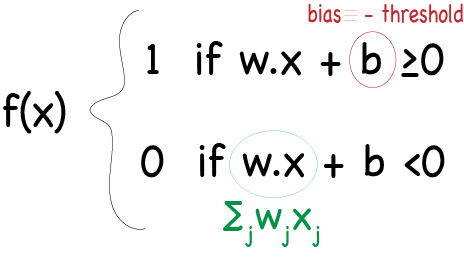One of the strongest point in this algorithm is that we can vary the weights and the bias to obtain distinct models of decision-making. We can assign more weight to those inputs so that if they are positive, it will favor our desired output. Also, because the bias can be understood as a measure of how difficult or easy is to output 1, we can drop or raise its value if we want to make more or less likely the desired output to happen. If we pay attention to the formula, we can observe that a big positive bias will make it very easy to output 1; however a very negative bias will make the task of output 1 very unlikely.

In consequence, a perceptron can analyze different evidence or data and make a decision according to the set preferences. It is possible, in fact, to create more complex networks including more layers of perceptrons where every layer takes the output of the previous one and weights it and make a more and more complex decisions.

What wait a minute: If perceptrons can do a good job in making complex decisions, why do we need other type of neuron? One of the disadvantages about a network containing perceptrons is that small changes in weights or bias, even in only one perceptron, can severely change our output going from 0 to 1 or vice versa. What we really want is to be able to gradually change the behaviour of our network by introducing small modifications in the weights or bias. Here is where a more modern type of neuron come in handy (Nowadays its use has been replaced by other types like Tanh and lately, by ReLu): Sigmoid neurons. The main difference between a sigmoid neuron and a perceptron is that the input and the output can be any continuous value between 0 and 1. The output is obtained after applying the sigmoid function to the inputs considering the weights, w, and the bias, b. To visualize it better, we can write the following: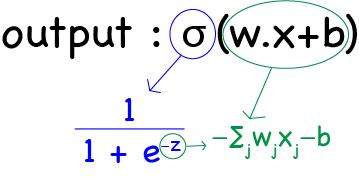So, the formula of the output is: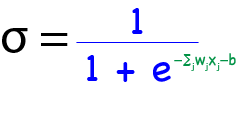If we perform a mathematical analysis of this function, we can make a graph of our function _σ _, shown below, and conclude that when z is large and positive the function reaches its maximum asymptotic value of 1; however, if z is large and negative, the function reaches its minimum asymptotic value of 0. Here is where the sigmoid function becomes very interesting because it is with moderate values of z that the function takes a smooth and close to linear shape. In this interval, small changes in weights (Δwj) or in bias (Δbj) will generate small changes in the output; the desired behaviour that we were looking for as an improvement from a perceptron.

We know that the derivative of a function is the measure of the rate at which the value y changes with respect to the change of the variable x. In this case, the variable y is our output and the variable x is a function of the weights and the bias. We can take advantage of this and calculate the change in the output using the derivatives, and particularly, the partial derivatives (with respect to w and with respect to b). You can read this post to follow the calculations but in the case of sigmoid function, the derivative will be reduce to calculate: f(z)*(1-f(z)).

Here it’s a simple code that can be used to model a sigmoid function:

We have just explain the functioning of every neuron in our network, but now, we can examine how the rest of the it works. A neural networks in which the output from one layer is used as the input of the next layer is called feedforward, particularly because there is no loops involved and the information is only pass forward and never back.

Suppose that we have a training set and we want to use a 3-layer neural network, in which we also use the sigmoid neuron we saw above, to predict a certain feature. Taking what we explain about the structure of a neural network, weights and bias need to be first assigned to the connections between neurons in one layer and the next layer. Normally, the biases and weights are all initialized randomly in a synapsis matrix. If we are coding the neural network in python, we can use the Numpy function np.random.random generating a Gaussian distributions (where mean is equal to 0 and standard deviation to 1) to have a place to start learning.

After that, we will build the neural network starting with the Feedforward step to calculate the predicted output; in other words, we just need to build the different layers involved in the network:

• layer0 is the input layer; our training set read as a matrix (We can called it X)
• layer1 is obtained by apply the activation function a’ = σ(w.X+b), in our case, performing the dot multiplication between input layer0 and the synapsis matrix syn0
• layer2is the output layer obtained by the dot multiplication between layer1 and its synapsis syn1

We will also need to iterate over the training set to let the network learn (we will see this later). In order to do so, we will add a for ** ** loop.

Until now, we have create the basic structure of the neural network: the different layers, the weights and bias of the connection between the neurons, and the sigmoid function. But none of this explains how the neural network can do such a good job in predicting patterns in a dataset. And this is what will take us to our last question.

#### WHY Neural Networks are able to learn?

The main strength of machine learning algorithms is their ability to learn and improve every time in predicting an output. But what does it mean that they can learn? In the context of neural networks, it implies that the weights and biases that define the connection between neurons become more precise; this is, eventually, the weights and biases are selected such as the output from the network approximates the real value y(x) for all the training inputs.

So, how do we quantify how far our prediction is from our real value in order for us to know if we need to keep searching for more precise parameters? For this aim, we need to calculate an error or in other words, define a cost function (Cost function is not other thing that the error in predicting the correct output that our network has; in other terms, it is the difference between the expected and the predicted output). In neural networks, the most commonly used one is the quadratic cost function, also called mean squared error, defined by the formula: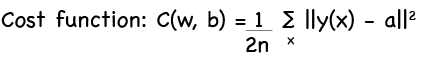This function is preferred over the linear error due to the fact that in neural networks small changes in weights and biases do not produces any change in the number of correct outputs; so using a quadratic function where big differences have more effect on the cost function than small ones help figuring out how to modify these parameters.

On the other hand, we can see that our cost function become smaller as the output is closer to the real value y, for all training inputs. The main goal of our algorithm is to minimize this cost function by finding a set of weights and biases to make it as small as possible. And the main tool to achieve this goal is an algorithm called Gradient Descent.

Then, the next question that we should answer is how we can minimize the cost function. From calculus, we know that a function can have global maximum and/or minimum, that is, where the function achieves the maximum or minimum value that it can have. We also know that one way to obtained that point is calculating derivatives. However, it is easy to calculate when we have a function with two variables but in the case of neural network, they include a lot of variables which make this computation quite impossible to make.

Instead, let’s take a look at the graph below of a random function: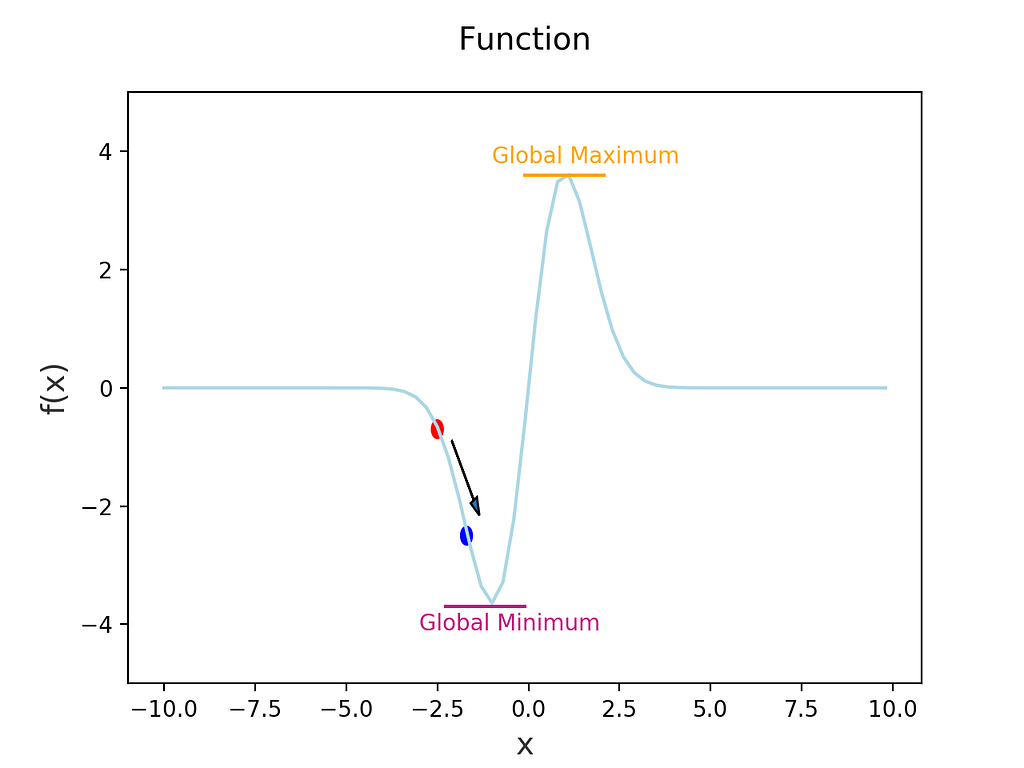We can see that this function has a global minimum. We could, as we said before, compute the derivatives to calculate where the minimum is located or we could take another approach. We can start in a random point and try to make a small move in the direction of the arrow, we would mathematically speaking, move Δx in the direction x and Δy in the direction of y, and calculate the change in our function ΔC. Because the rate of change in a direction is the derivative of a function, we could express the change in the function as: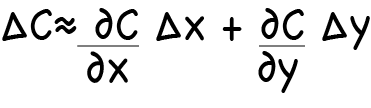Here, we will take the definition from calculus of the gradient of a function: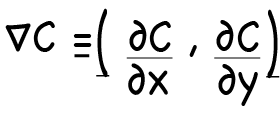Now, we can rewrite the change in our function as: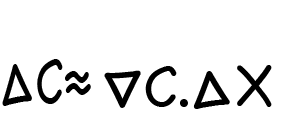So now, we can see what happens with cost function when we choose a certain change in our parameters. The amount that we choose to move in any direction is called learning rate, and it is what define how fast we move towards the global minimum. If we choose a very small number, we will need to make a too many moves to reach this point; however, if we choose a very big number, we are at risk of passing the point and never be able to reach it. So the challenge is to choose the learning rate small enough. After choosing the learning rate, we can update our weights and biases and make another move; process that we repeat in each iteration.

So, in few words, the gradient descent works by computing the gradient ∇C repeatedly, and then updating the weights and biases, and trying to find the correct values that minimize, in that way, the cost of function. And this is how the neural network learns.

Sometimes, calculating the gradient can be very complex. There is, however, a way to speed up this calculations called stochastic gradient descent. This works by estimating the gradient ∇C by computing instead the gradient for a small sample of randomly chosen training inputs. Then, this small samples are average to get a good estimate of the true gradient, speeding up gradient descent, and thus learning faster.

But wait a second? How do we compute the gradient of the cost function? Here is where another algorithm makes an entry: Backpropagation. The goal of this algorithm is to compute the partial derivatives of the cost function with respect to any weight w and any bias b; in practice, this means calculating the error vectors starting from the final layer and then, propagating this back to update the weights and biases. The reason why we need to go back is that the cost is a function of the output of our network. There are several calculations and errors that we need to compute whose formula are given by the backpropagation algorithm: 1) Output error (δL) related to the element wise (⦿) product of the gradient (▽C) by the derivative of activation function (σ′(z)), 2) error of one layer (ẟl) in terms of the error in the next layer related to the transpose matrix of the weights (Wl+1) multiplied by the error of the next layer (ẟl+1) and the element wise multiplication of the derivative of activation function, 3) rate of change of the cost with respect to any bias in the network: this means that the partial derivative of C with respect to any bias (∂C/∂bj) is equal to the error ẟl, 4) rate of change of the cost with respect to any weight in the network: meaning that the partial derivative of C with respect to any weight (∂C/∂wj) is equal to the error (ẟl) multiplied by activation of the neuron input. These last two calculation constitute the gradient of the cost function. Here, we can observe the formulas.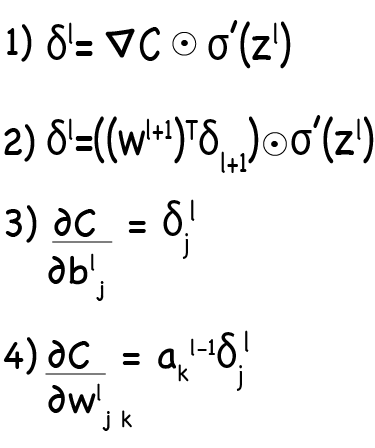The backpropagation algorithm calculates the gradient of the cost function for only one single training example. As a consequence, we need to combine backpropagation with a learning algorithm, for instance stochastic gradient descent, in order to compute the gradient for all the training set.

Now, how do we apply this to our neural network in python?. Here, we can see step by step the calculations:

#### Let’s wrap up everything…

Now we can put all of these formulas and concepts that we have seen in terms of an algorithm to see how we can implement this:

• INPUT : We input a set of training examples and we set the activation a that correspond for the input layer_._
• FEEDFORWARD : For each layer, we compute the function z = w . a + b, being a = σ(z)
• OUTPUT ERROR : We compute the output error by using the formula #1 cited above.
• BACKPROPAGATION : Now we backpropagate the error; for each layer, we compute the formula #2 cited above.
• OUTPUT : We calculate the gradient descent with respect to any weight and bias by using the formulas #3 and #4.

Of course, that there are more concepts, implementations and improvements that can be done to neural networks, which can become more and more widely used and powerful through the last years. But I hope this information can give you a hint on what a neural network is, how it works and learns using gradient descent and backpropagation.

References: